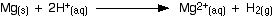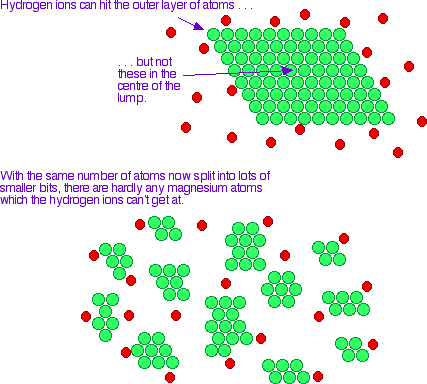Need help? Email help@shout.education

# The Effect of Surface Area on Rates of Reaction

This page describes and explains the effect of changing the surface area of a solid on the rate of a reaction it is involved in. This applies to reactions involving a solid and a gas, or a solid and a liquid. It includes cases where the solid is acting as a catalyst.

## The Facts

### What Happens?

The more finely divided the solid is, the faster the reaction happens. A powdered solid will normally produce a faster reaction than if the same mass is present as a single lump. The powdered solid has a greater surface area than the single lump.

Note: Why normally? What exceptions can there be?

Imagine a case of a very fine powder reacting with a gas. If the powder was in one big heap, the gas may not be able to penetrate it. That means that its effective surface area is much the same as (or even less than) it would be if it were present in a single lump.

A small heap of fine magnesium powder tends to burn rather more slowly than a strip of magnesium ribbon, for example.

### Some Examples

#### Calcium carbonate and hydrochloric acid

In the lab, powdered calcium carbonate reacts much faster with dilute hydrochloric acid than if the same mass was present as lumps of marble or limestone.

\text{CaCO}_{3(s)} + 2\text{HCl}_{(aq)} \longrightarrow \text{CaCl}_{2(aq)} + \text{H}_2\text{O}_{(l)} + \text{CO}_{s(g)}

#### The catalytic decomposition of hydrogen peroxide

This is another familiar lab reaction. Solid manganese(IV) oxide is often used as the catalyst. Oxygen is given off much faster if the catalyst is present as a powder than as the same mass of granules.

2\text{H}_2\text{O}_{2(aq)} \xrightarrow{\text{MnO}_{2(s)}} 2\text{H}_2\text{O}_{(l)} + \text{O}_{2(g)}

#### Catalytic converters

Catalytic converters use metals like platinum, palladium and rhodium to convert poisonous compounds in vehicle exhausts into less harmful things. For example, a reaction which removes both carbon monoxide and an oxide of nitrogen is:

2\text{CO}_{(g)} + 2\text{NO}_{(g)} \longrightarrow 2\text{CO}_{2(g)} + \text{N}_{2(g)}

Because the exhaust gases are only in contact with the catalyst for a very short time, the reactions have to be very fast. The extremely expensive metals used as the catalyst are coated as a very thin layer onto a ceramic honeycomb structure to maximise the surface area.

## The Explanation

You are only going to get a reaction if the particles in the gas or liquid collide with the particles in the solid. Increasing the surface area of the solid increases the chances of collision taking place.

Imagine a reaction between magnesium metal and a dilute acid like hydrochloric acid. The reaction involves collision between magnesium atoms and hydrogen ions.Increasing the number of collisions per second increases the rate of reaction.